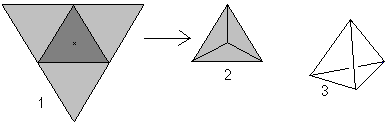# Java Program to Calculate Surface Area of a Regular Tetrahedron

In this program, you will learn to to Calculate Surface Area of a Tetrahedron in Java. A Tetrahedron is simply a pyramid with a triangular base. It is a solid object with four triangular faces, three on the sides or lateral faces, one on the bottom or the base and four vertices or corners. If the faces are all congruent equilateral triangles, then the tetrahedron is called regular.http://mathematische-basteleien.de/

A tetrahedron is a three-dimensional figure with four equilateral triangles. If you lift up three triangles (1), you get the tetrahedron in top view (2). Generally it is shown in perspective (3).

#### The Surface area of  Tetrahedron can be found by using the formula :

The area of the base and the lateral faces form the surface O. There is O=4*A (triangle) = sqrt(3)*(side*side)

#### When you run the program, the output will be:

`Surface Area of Tetrahedron is 27.712812921102035`